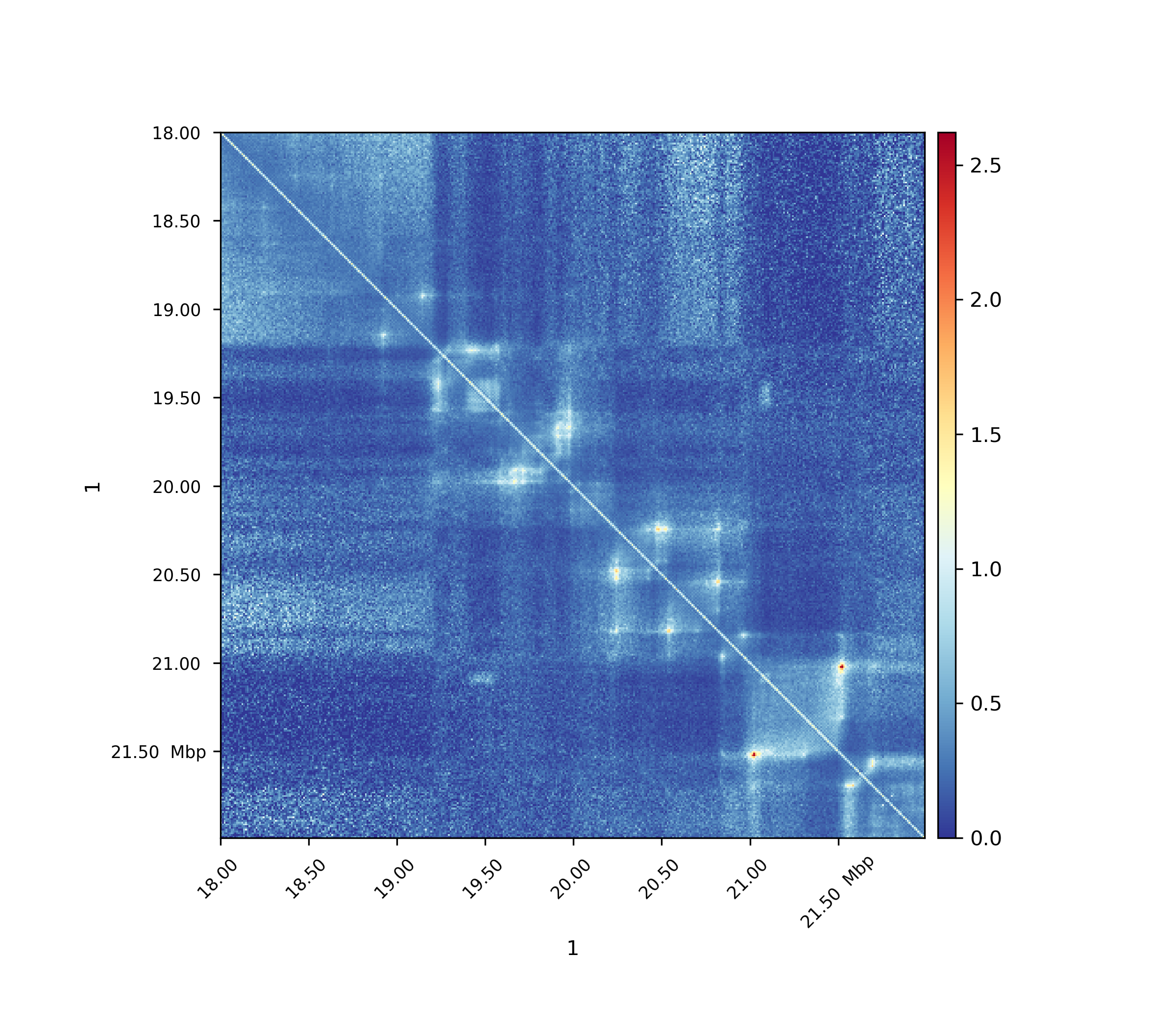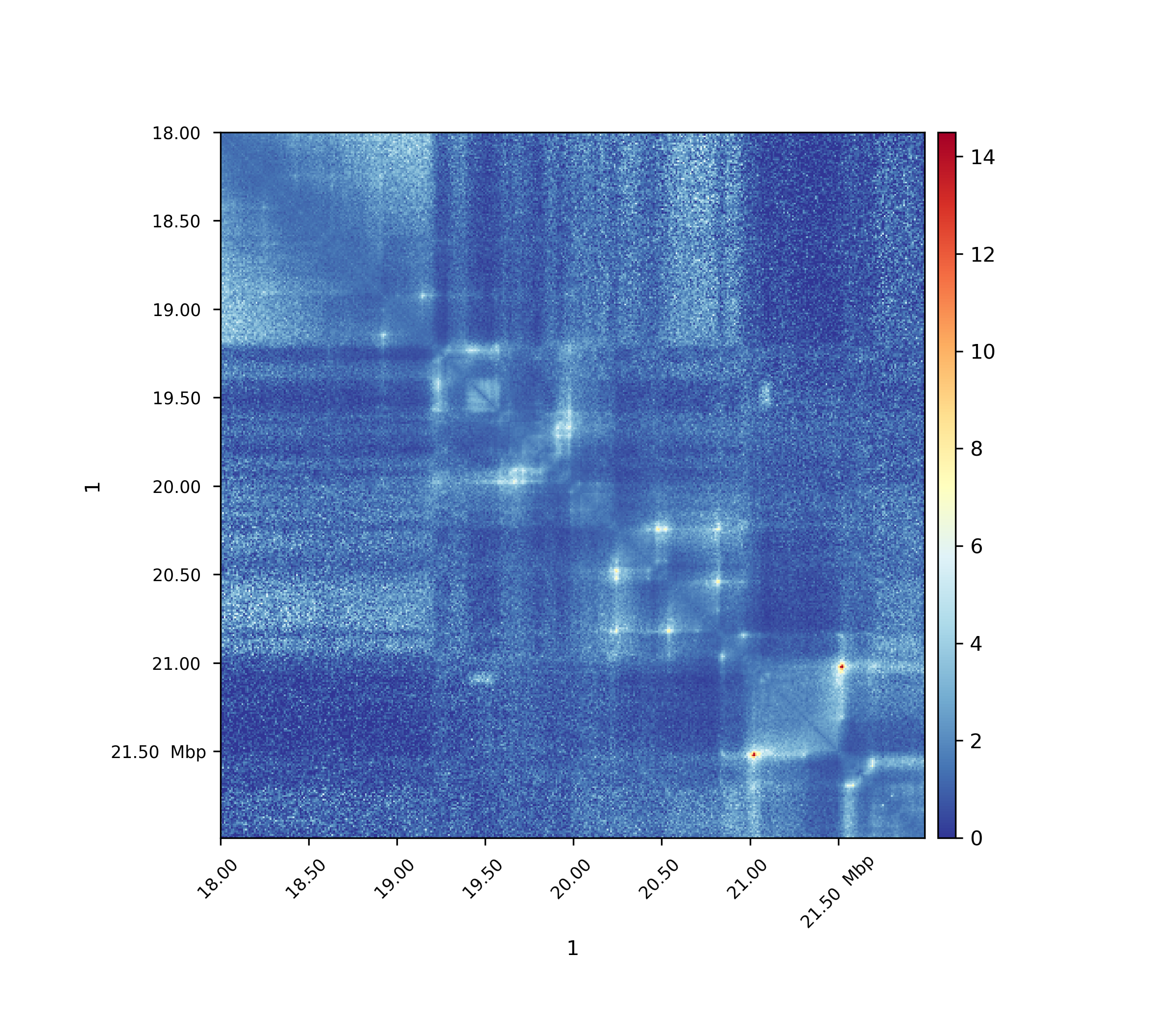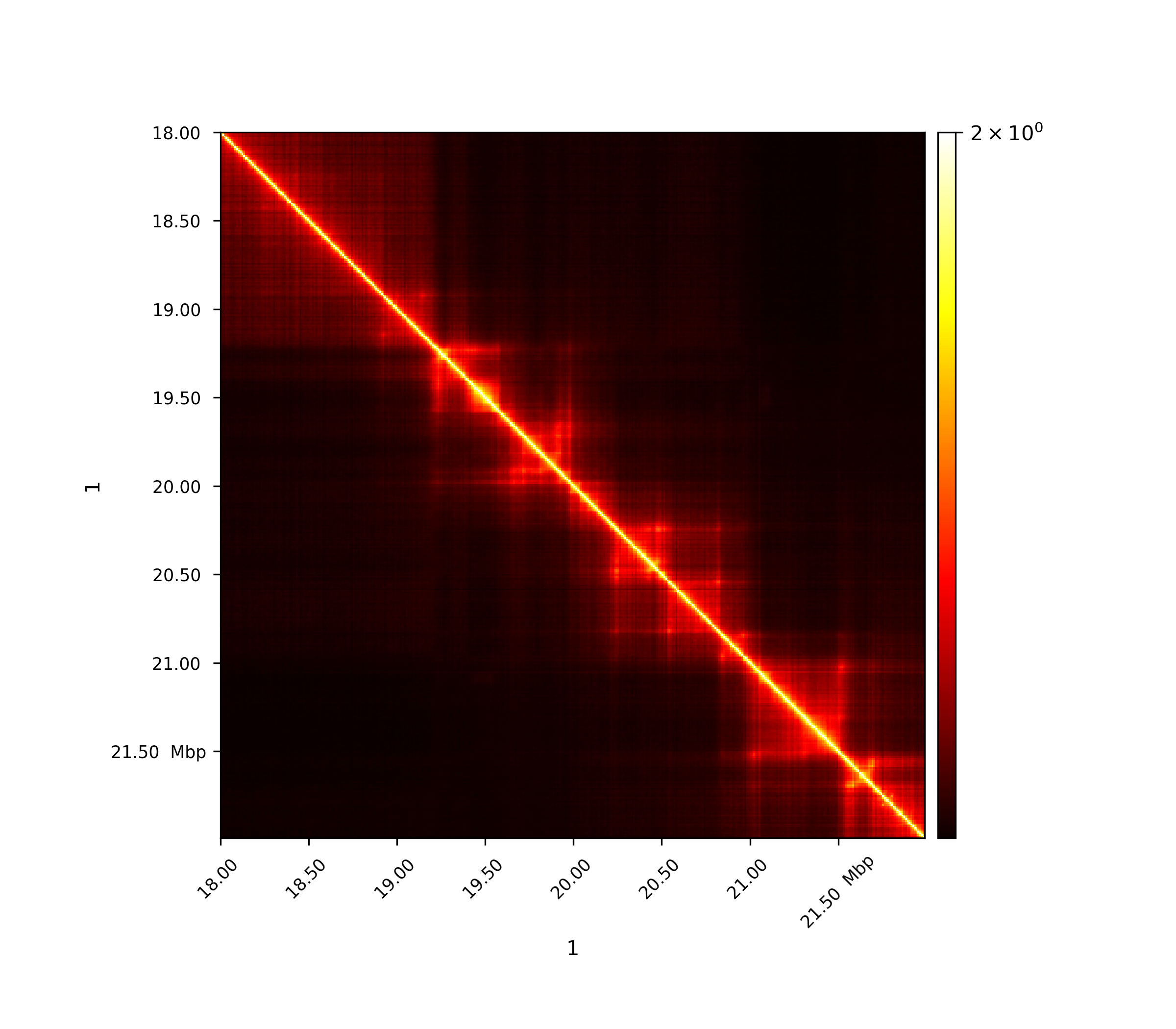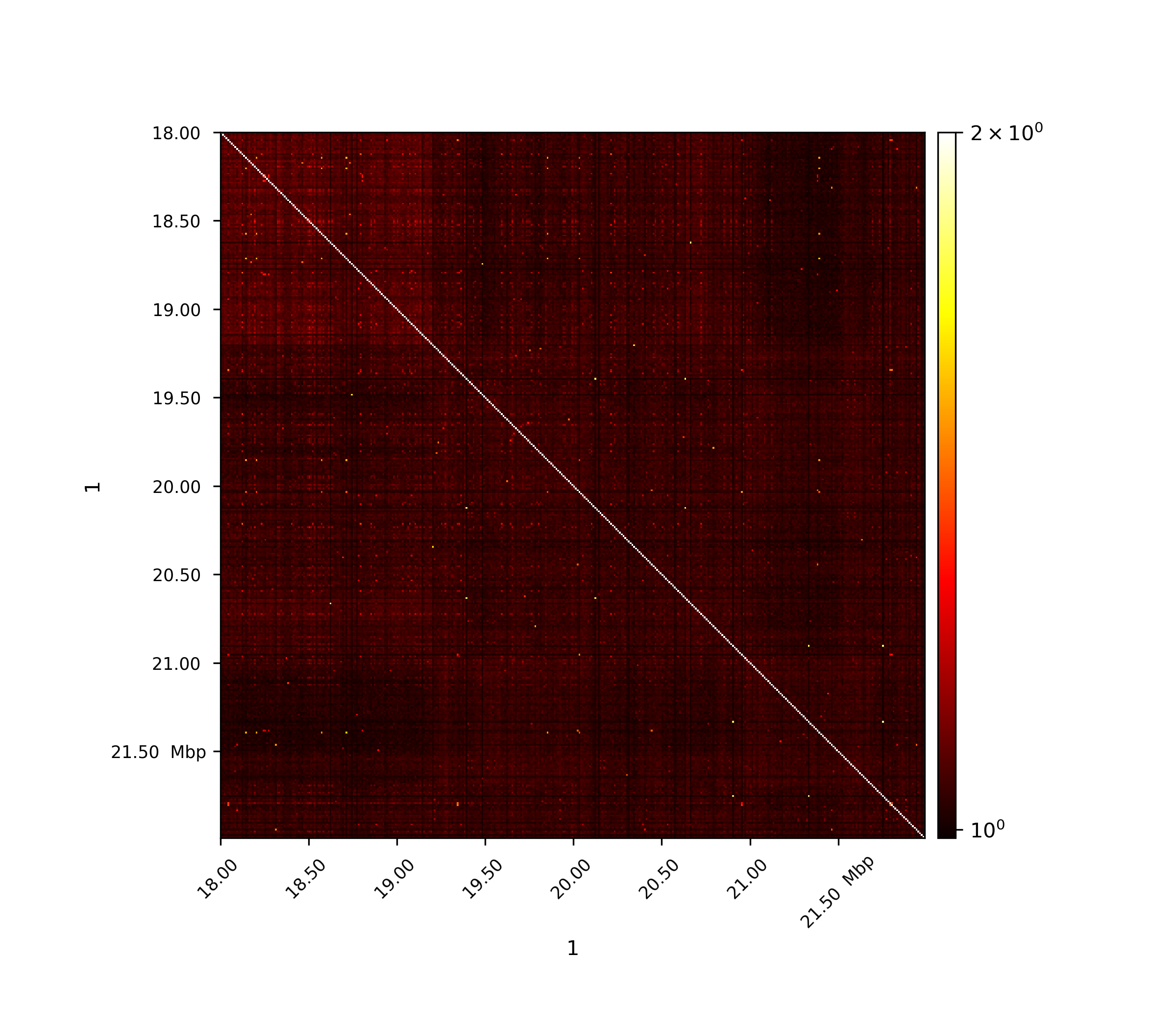# hicTransform¶

Converts the (interaction) matrix to different types of obs/exp, pearson or covariance matrix.

usage: hicTransform --matrix MATRIX --outFileName OUTFILENAME
[--method {obs_exp,obs_exp_lieberman,obs_exp_non_zero,obs_exp_norm,pearson,covariance}]
[--chromosomes CHROMOSOMES [CHROMOSOMES ...]]
[--perChromosome] [-help] [--version]


## Required arguments¶

--matrix, -m

input file. The computation is done per chromosome.

--outFileName, -o

File name to save the exported matrix.

## Optional arguments¶

--method, -me

Possible choices: obs_exp, obs_exp_lieberman, obs_exp_non_zero, obs_exp_norm, pearson, covariance

Transformation method to use for input matrix. Transformation is computed per chromosome.obs_exp computes the expected matrix as the sum per genomic distance j divided by maximal possible contacts: sum(diagonal(j) / number of elements in diagonal(j) obs_exp_lieberman computes the expected matrix as the sum per genomic distance j divided by the : sum(diagonal(j) / (length of chromosome - j))obs_exp_non_zero computes the expected matrix as the sum per genomic distance j divided by sum of non-zero contacts: sum(diagonal(j) / number of non-zero elements in diagonal(j)obs_exp_norm computes the expected matrix for exp_i,j: sum(diagonal(i-j)) * sum(row(j)) * sum(row(i)) / sum(matrix)pearson computes the Pearson correlation matrix on the input matrix: Pearson_i,j = C_i,j / sqrt(C_i,i * C_j,j) and C is the covariance matrixcovariance computes the Covariance matrix on the input matrix: Cov_i,j = E[M_i, M_j] - my_i * my_j where M is the input matrix and my the mean.

Default: “obs_exp”

--chromosomes

List of chromosomes to be included in the computation.

--perChromosome, -pc

Each chromosome is processed individually, inter-chromosomal interactions are ignored. Option not valid for obs_exp_lieberman.

Default: False

--version

show program’s version number and exit

# Background¶

hicTransform transforms a given input matrix to a matrix with the defined method applied on.

• obs_exp

• obs_exp_norm

• obs_exp_lieberman

• obs_exp_non_zero

• pearson

• covariance

All expected values are computed per genomic distances.

## Usage¶

\$ hicTransform -m matrix.cool --method obs_exp -o obs_exp.cool


For all images data from Rao 2014 was used.

## Observed / Expected¶

All values, including non-zero values, are used to compute the expected values per genomic distance.

$exp_{i,j} = \frac{ \sum diagonal(|i-j|) }{|diagonal(|i-j|)|}$## Observed / Expected norm¶

The expected matrix is computed in the same way Homer software computes it with the option ‘-norm’ set, to conserve this reference, HiCExplorer names this expected matrix computation ‘norm’. The usage of this expected value computation gives better results for A/B compartment computation for drosophila genomes.

$exp_{i,j} = \frac{ \sum diagonal(|i-j|) * \sum row(j) * \sum row(i) }{ \sum matrix }$## Observed / Expected lieberman¶

The expected matrix is computed in the way Lieberman-Aiden used it in the 2009 publication, it is quite similar to obs/exp matrix computation.

$exp_{i,j} = \frac{ \sum diagonal(|i-j|) } {(length\ of\ chromosome\ - |i-j|))}$## Observed / Expected non zero¶

Only non-zero values are used to compute the expected values per genomic distance, i.e. only non-zero values are taken into account for the denominator.

$exp_{i,j} = \frac{ \sum diagonal(i-j) }{ number\ of\ non-zero\ elements\ in\ diagonal(|i-j|)}$## Pearson correlation matrix¶

$Pearson_{i,j} = \frac {C_{i,j} }{ \sqrt{C_{i,i} * C_{j,j} }}$

C is the covariance matrixThe first image shows the Pearson correlation on the original interaction matrix, the second one shows the Person correlation matrix on an observed/expected matrix. A consecutive computation like this is used in the A/B compartment computation.

## Covariance matrix¶

$Cov_{i,j} = E[M_i, M_j] - \mu_i * \mu_j$

where M is the input matrix and $$\mu$$ the mean.